Anzeige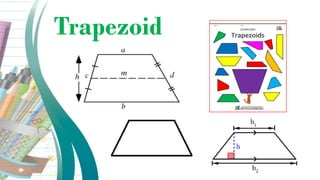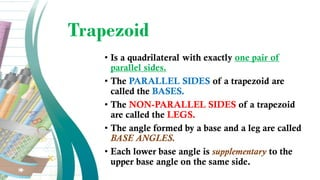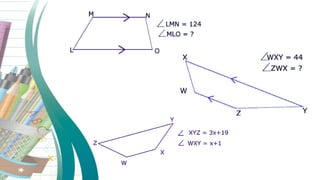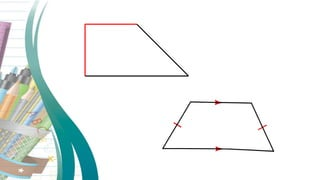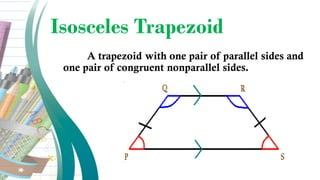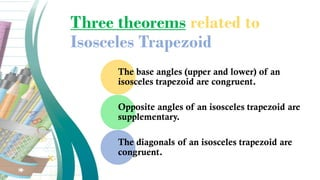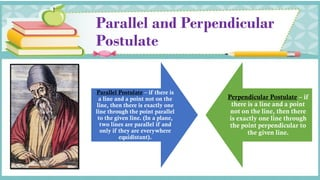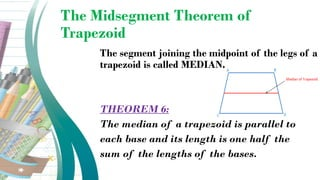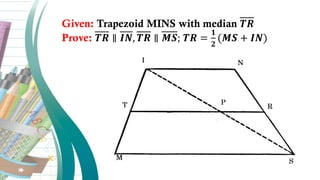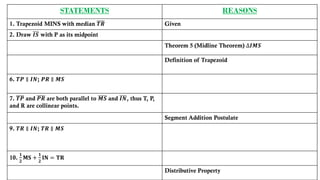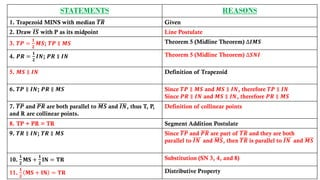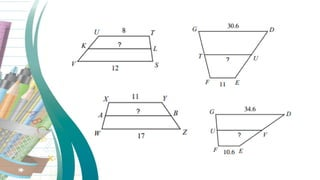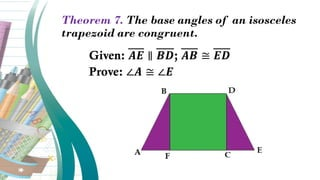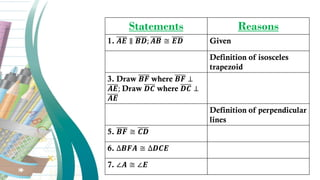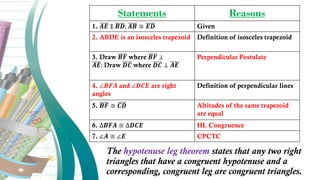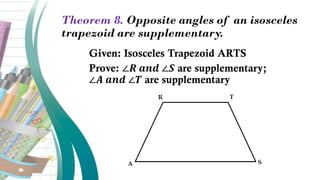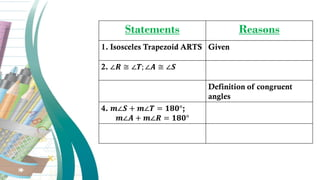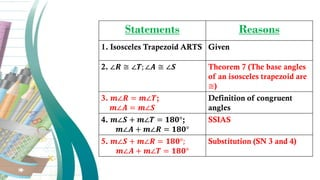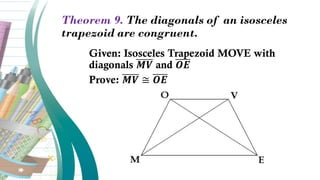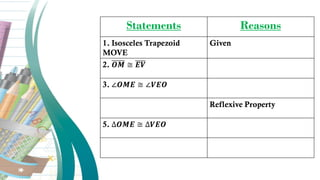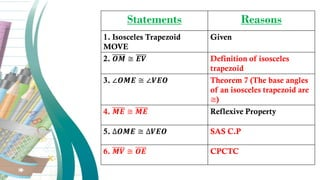1 von 23
Anzeige

### Trapezoid-and-Isosceles-Trapezoid-Theorems-6-9-1 (1).pdf

1. Trapezoid
2. Trapezoid • Is a quadrilateral with exactly one pair of parallel sides. • The PARALLEL SIDES of a trapezoid are called the BASES. • The NON-PARALLEL SIDES of a trapezoid are called the LEGS. • The angle formed by a base and a leg are called BASE ANGLES. • Each lower base angle is supplementary to the upper base angle on the same side.
3. Isosceles Trapezoid A trapezoid with one pair of parallel sides and one pair of congruent nonparallel sides.
4. Three theorems related to Isosceles Trapezoid The base angles (upper and lower) of an isosceles trapezoid are congruent. Opposite angles of an isosceles trapezoid are supplementary. The diagonals of an isosceles trapezoid are congruent.
5. Parallel and Perpendicular Postulate Parallel Postulate – if there is a line and a point not on the line, then there is exactly one line through the point parallel to the given line. (In a plane, two lines are parallel if and only if they are everywhere equidistant). Perpendicular Postulate – if there is a line and a point not on the line, then there is exactly one line through the point perpendicular to the given line.
6. The Midsegment Theorem of Trapezoid The segment joining the midpoint of the legs of a trapezoid is called MEDIAN. THEOREM 6: The median of a trapezoid is parallel to each base and its length is one half the sum of the lengths of the bases.
7. Given: Trapezoid MINS with median 𝑻𝑹 Prove: 𝑻𝑹 ∥ 𝑰𝑵, 𝑻𝑹 ∥ 𝑴𝑺; 𝑻𝑹 = 𝟏 𝟐 (𝑴𝑺 + 𝑰𝑵)
8. STATEMENTS REASONS 1. Trapezoid MINS with median 𝑻𝑹 Given 2. Draw 𝑰𝑺 with P as its midpoint Theorem 5 (Midline Theorem) ∆𝑰𝑴𝑺 Definition of Trapezoid 6. 𝑻𝑷 ∥ 𝑰𝑵; 𝑷𝑹 ∥ 𝑴𝑺 7. 𝑻𝑷 and 𝑷𝑹 are both parallel to 𝑴𝑺 and 𝑰𝑵, thus T, P, and R are collinear points. Segment Addition Postulate 9. 𝑻𝑹 ∥ 𝑰𝑵; 𝑻𝑹 ∥ 𝑴𝑺 10. 𝟏 𝟐 𝐌𝐒 + 𝟏 𝟐 𝐈𝐍 = 𝐓𝐑 Distributive Property
9. STATEMENTS REASONS 1. Trapezoid MINS with median 𝑻𝑹 Given 2. Draw 𝑰𝑺 with P as its midpoint Line Postulate 3. 𝑻𝑷 = 𝟏 𝟐 𝑴𝑺; 𝑻𝑷 ∥ 𝑴𝑺 Theorem 5 (Midline Theorem) ∆𝑰𝑴𝑺 4. 𝑷𝑹 = 𝟏 𝟐 𝑰𝑵; 𝑷𝑹 ∥ 𝑰𝑵 Theorem 5 (Midline Theorem) ∆𝑺𝑵𝑰 5. 𝑴𝑺 ∥ 𝑰𝑵 Definition of Trapezoid 6. 𝑻𝑷 ∥ 𝑰𝑵; 𝑷𝑹 ∥ 𝑴𝑺 Since 𝑻𝑷 ∥ 𝑴𝑺 and 𝑴𝑺 ∥ 𝑰𝑵, therefore 𝑻𝑷 ∥ 𝑰𝑵 Since 𝑷𝑹 ∥ 𝑰𝑵 and 𝑴𝑺 ∥ 𝑰𝑵, therefore 𝑷𝑹 ∥ 𝑴𝑺 7. 𝑻𝑷 and 𝑷𝑹 are both parallel to 𝑴𝑺 and 𝑰𝑵, thus T, P, and R are collinear points. Definition of collinear points 8. TP + PR = TR Segment Addition Postulate 9. 𝑻𝑹 ∥ 𝑰𝑵; 𝑻𝑹 ∥ 𝑴𝑺 Since 𝑻𝑷 and 𝑷𝑹 are part of 𝑻𝑹 and they are both parallel to 𝑰𝑵 and 𝑴𝑺, then 𝑻𝑹 is parallel to 𝑰𝑵 and 𝑴𝑺 10. 𝟏 𝟐 𝐌𝐒 + 𝟏 𝟐 𝐈𝐍 = 𝐓𝐑 Substitution (SN 3, 4, and 8) 11. 𝟏 𝟐 𝐌𝐒 + 𝐈𝐍 = 𝐓𝐑 Distributive Property
10. Theorem 7. The base angles of an isosceles trapezoid are congruent. Given: 𝑨𝑬 ∥ 𝑩𝑫; 𝑨𝑩 ≅ 𝑬𝑫 Prove: ∠𝑨 ≅ ∠𝑬
11. Statements Reasons 1. 𝑨𝑬 ∥ 𝑩𝑫; 𝑨𝑩 ≅ 𝑬𝑫 Given Definition of isosceles trapezoid 3. Draw 𝑩𝑭 where 𝑩𝑭 ⊥ 𝑨𝑬; Draw 𝑫𝑪 where 𝑫𝑪 ⊥ 𝑨𝑬 Definition of perpendicular lines 5. 𝑩𝑭 ≅ 𝑪𝑫 6. ∆𝑩𝑭𝑨 ≅ ∆𝑫𝑪𝑬 7. ∠𝑨 ≅ ∠𝑬
12. Statements Reasons 1. 𝑨𝑬 ∥ 𝑩𝑫; 𝑨𝑩 ≅ 𝑬𝑫 Given 2. ABDE is an isosceles trapezoid Definition of isosceles trapezoid 3. Draw 𝑩𝑭 where 𝑩𝑭 ⊥ 𝑨𝑬; Draw 𝑫𝑪 where 𝑫𝑪 ⊥ 𝑨𝑬 Perpendicular Postulate 4. ∠𝑩𝑭𝑨 and ∠𝑫𝑪𝑬 are right angles Definition of perpendicular lines 5. 𝑩𝑭 ≅ 𝑪𝑫 Altitudes of the same trapezoid are equal 6. ∆𝑩𝑭𝑨 ≅ ∆𝑫𝑪𝑬 HL Congruence 7. ∠𝑨 ≅ ∠𝑬 CPCTC The hypotenuse leg theorem states that any two right triangles that have a congruent hypotenuse and a corresponding, congruent leg are congruent triangles.
13. Theorem 8. Opposite angles of an isosceles trapezoid are supplementary. Given: Isosceles Trapezoid ARTS Prove: ∠𝑹 𝒂𝒏𝒅 ∠𝑺 are supplementary; ∠𝑨 𝒂𝒏𝒅 ∠𝑻 are supplementary
14. Statements Reasons 1. Isosceles Trapezoid ARTS Given 2. ∠𝑹 ≅ ∠𝑻; ∠𝑨 ≅ ∠𝑺 Definition of congruent angles 4. 𝒎∠𝑺 + 𝒎∠𝑻 = 𝟏𝟖𝟎°; 𝒎∠𝑨 + 𝒎∠𝑹 = 𝟏𝟖𝟎°
15. Statements Reasons 1. Isosceles Trapezoid ARTS Given 2. ∠𝑹 ≅ ∠𝑻; ∠𝑨 ≅ ∠𝑺 Theorem 7 (The base angles of an isosceles trapezoid are ≅) 3. 𝒎∠𝑹 = 𝒎∠𝑻; 𝒎∠𝑨 = 𝒎∠𝑺 Definition of congruent angles 4. 𝒎∠𝑺 + 𝒎∠𝑻 = 𝟏𝟖𝟎°; 𝒎∠𝑨 + 𝒎∠𝑹 = 𝟏𝟖𝟎° SSIAS 5. 𝒎∠𝑺 + 𝒎∠𝑹 = 𝟏𝟖𝟎°; 𝒎∠𝑨 + 𝒎∠𝑻 = 𝟏𝟖𝟎° Substitution (SN 3 and 4)
16. Theorem 9. The diagonals of an isosceles trapezoid are congruent. Given: Isosceles Trapezoid MOVE with diagonals 𝑴𝑽 and 𝑶𝑬 Prove: 𝑴𝑽 ≅ 𝑶𝑬
17. Statements Reasons 1. Isosceles Trapezoid MOVE Given 2. 𝑶𝑴 ≅ 𝑬𝑽 3. ∠𝑶𝑴𝑬 ≅ ∠𝑽𝑬𝑶 Reflexive Property 5. ∆𝑶𝑴𝑬 ≅ ∆𝑽𝑬𝑶
18. Statements Reasons 1. Isosceles Trapezoid MOVE Given 2. 𝑶𝑴 ≅ 𝑬𝑽 Definition of isosceles trapezoid 3. ∠𝑶𝑴𝑬 ≅ ∠𝑽𝑬𝑶 Theorem 7 (The base angles of an isosceles trapezoid are ≅) 4. 𝑴𝑬 ≅ 𝑴𝑬 Reflexive Property 5. ∆𝑶𝑴𝑬 ≅ ∆𝑽𝑬𝑶 SAS C.P 6. 𝑴𝑽 ≅ 𝑶𝑬 CPCTC
Anzeige